Support the Monkey! Tell All your Friends and TeachersHome MonkeyNotes Printable Notes Digital Library Study Guides Study Smart Parents Tips College Planning Test Prep Fun Zone Help / FAQ How to Cite New Title Request

2.3 Functions of General Angles or t -Ratios

Draw a unit circle (circle of radius 1 unit). Let O be the centre of this unit circle. Set a rectangular right-hand co-ordinate system, taking O as its origin (see fig).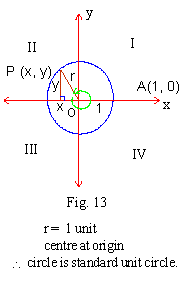Let the circle cut the x-axis at A º (1,0). Rotate OA around O (counter clockwise or clock wise) reaching OP and forming an angle of measure q .

Obviously OP = 1 unit.

Let P º (x, y) then we define the following

1. sin q = y / r or y / 1 = y (\ r = 1)

2. cos q = x / r or x / 1 = x (\ r = 1)

3. tan q = y / x provided x ¹ 0

4. cot q = x /y provided y ¹ 0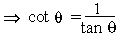5. csc q = r /y = 1/y provided
y ¹ 0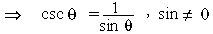6. sec q = r/x = 1/x provided x ¹ 0Your browser does not support the IFRAME tag.

Note : Whenever we wish to find the values of t- ratios of standard angles or prove some result, we shall say "Draw a unit standard circle and let angle q be in standard position." This will automatically mean that the initial side of the angle is along the x-axis, vertex being at the origin.

The Signs Of Trigonometric Ratios

Since r is always positive, the signs of the functions in various quadrant depend upon the signs of x and y. To determine these signs one may visualize the angle in standard position.

1) If P(x, y) be in the 1st quadrant both x and y are positive. Hence all t-ratios are positive.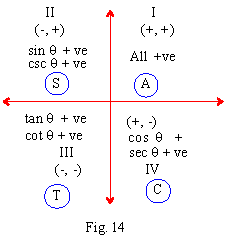2) If P (x, y) be in the IInd quadrant x is negative and y is positive.

\ sin q = y / r , csc q = r / y are positive

cos q = x / r, sec q = r / x, tan q = y / x and cot q = x / y are all negative

3) If P (x,y) be in the IIIrd quadrant, both x and y are negative.
Hence sin q y /r , cos q = x / r, csc q = r / y

and sec q r / x are all negative whereas tan q = y / x and cot q = x / y are positive.

4) If P(x, y) be in the IVth quadrant, x is positive but y is negative.

Hence cos q = x / r and sec q = r /x are positive but sin q = y / r, csc q = r / y, tan q = y /x and cot q = x/y are all negative.

A crude aid to remember the signs is the four letter acronym (phrase) ASTC which stands for All Silver Tea Cups or "Arizona State Teacher's College" or any other four worded phrase that will help you to remember the relationships.

Note:
 A S T C ¯ ¯ ¯ ¯ All are +ve In Ist quad. sine and csc. are +ve in II quad. tan and cot are +ve in III quad cos and sec are + ve in IV quad

For these angles the terminal side of an angle coincides with one of the co-ordinate axes. Therefore point P(x, y) on the terminal side has either x = 0, y ¹ 0 or x ¹ 0, y = 0. In either case, two of the six t-ratios are not defined. For instance, for angle 00 terminal side coincides with +ve of x-axis and its ordinate i.e y = 0. Now cot q = x / y and csc q = r / y, having y = 0 in the denominator are not defined. Some authors write this as cot 00 = ± ¥ or simply cot 00 = ¥ .

The t- ratios of the quadrantal angles are :-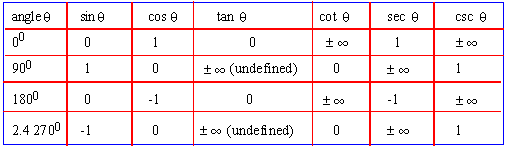Index

2.1 Trigonometric Ratio of Acute Angles
2.2 Fundamental Relation between the trigonometric Ratios of an angle
2.3 Functions of General Angles or t Ratio
2.4 Tables of Trigonometric Function

Supplementary Problems

Chapter 3Search: All Products Books Popular Music Classical Music Video DVD Toys & Games Electronics Software Tools & Hardware Outdoor Living Kitchen & Housewares Camera & Photo Cell Phones Keywords: